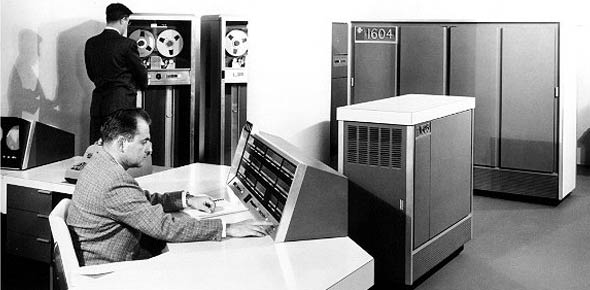# 2A533A Volume 4 CDC Test

30 Questions | Total Attempts: 37SettingsYou know

• 1.
What is the purpose of the very-high omnirange system?
• A.

Determine bearing to a station

• B.

Steering indications

• C.

Provides recommendations to the pilot for aircraft maneuvers

• D.

Alititude

• 2.
The basic operating principle of very-high frequency omnirange is phase comparing the?
• A.

30-Hertz (Hz) reference and 30-Hz variable signals

• B.

30-Hertz(Hz) reference and 9010-Hz variable signals

• C.

9010-Hertz(Hz) reference and 30-Hz variable signals

• D.

There is no phase comparing

• 3.
If the magnetic bearing to a very-high frequency omnirange station is 225 degrees, the phase difference between the 30-Hertz (Hz) reference and 30 (Hz) variable signal is?
• A.

15 degrees

• B.

90 degrees

• C.

45 degrees

• D.

60 degrees

• 4.
Very-high frequency omnirange aural (station identification) signals are transmitted?
• A.

Intervals of 5 micro-seconds

• B.

Continuously

• C.

Intervals of 5 seconds

• 5.
The instrument landing system localizer course width at a distance of 10 miles from the transmitter is normally?
• A.

5 degrees

• B.

15 degrees

• C.

35 degrees

• D.

60 degrees

• 6.
What frequency band is used to transmit instrument landing system glide slope information?
• A.

VHF

• B.

HF

• C.

UHF

• D.

IFF

• 7.
The instrument landing system marker beacon carrier frequency is?
• A.

75Hz

• B.

75 MHz

• C.

50 MHz

• D.

50Hz

• 8.
What microwave landing system outputs are provided to the pilot's and copilot's horizontal situation and attitude director indicators for approach and takeoff?
• A.

Steering indications

• B.

Airspeed indications

• C.

Groundspeed indications

• D.

Invisible indications

• 9.
On how many channels are the microwave landing systems receivers capable of operating?
• A.

200

• B.

20

• C.

40

• D.

5

• 10.
What is the frequency range of the microwave landing system receivers?
• A.

1020-1300 MHz

• B.

5031-5091 Hz

• C.

5031-5091 MHz

• D.

1020-1300 Hz

• 11.
Which antennas are shared by microwave landing system numbers 1 and 2?
• A.

1 and 2

• B.

2 and 3

• C.

1 and 4

• D.

3 and 4

• 12.
Which microwave landing system component allows for selection of system status?
• A.

Integrated display computer unit

• B.

EGI

• C.

MMR

• D.

Control Processor

• 13.
When an instrument landing system frequency is selected, the ARN-127 very-high frequency omnirange receiver parks the horizontal situation indicator bearing pointer at the?
• A.

4 o'clock position

• B.

5 o'clock position

• C.

6 o'clock position

• D.

7 o'clock position

• 14.
Which radial will the aircradt be positioned on if the aircraft is located north of the very-high frequency omnirange station?
• A.

2.5 degrees

• B.

210 degrees

• C.

90 degrees

• D.

000 degrees

• 15.
To get a FROM flag in view on the horizontal situation indicator when the magnetic bearing to the very-high frequency omnirange station is 75 degrees, the selected course should be manually set to?
• A.

2.5 degrees

• B.

210 degrees

• C.

90 degrees

• D.

000 degrees

• 16.
During instrument landing system operation, a full scale deflection of the horizontal situation indicator vertical needle indicates the aircraft is off course from the ruway centerline by?
• A.

2.5 degrees

• B.

210 degrees

• C.

90 degrees

• D.

000 degrees

• 17.
In instrument landing system operation, a deflection of two dots on the attitude director indicator glide slope deviation scale indicates the aircraft is?
• A.

.6 degrees off glide path

• B.

.3 degrees off glide path

• C.

.25 degrees off glide path

• D.

.5 degrees off glide path

• 18.
when instrument landing system marker beacon amber (orange) lamp illuminates, the pilot hears a?
• A.

300 Hz Tone

• B.

15 Hz Tone

• C.

1,300 Hz Tone

• D.

13 Hz Tone

• 19.
The total number of very-high frequency omnirange/instrument landing system (VOR/ILS)channels that can be selected by the AN/ARN-147 VOR/ILS control box is?
• A.

20

• B.

200

• C.

150

• D.

220

• 20.
If the very-high frequency omnirange station signal is lost or the receiver malfunctions, the ARN-147 system parks the bearing pointer at the?
• A.

3 o'clock position

• B.

4 o'clock position

• C.

5 o'clock position

• D.

6 o'clock postion

• 21.
In very-high frequency omnirange(VOR) mode, what information is sent to the horizontal situation indicator by the ARN-147 VOR/localizer receiver?
• A.

UP/down deviation, flag alarm, and TO/From

• B.

Up/Down deviation, Left/Right Deviation, Flag Alarm

• C.

Left/right deviation, flag alarm, and TO/FROM

• 22.
What glide slope information is provided to the attitude director indicator when the AN/ARN-127 is operating in the instrument landing system mode?
• A.

UP/down deviations

• B.

Left/Right deviations

• C.

To/From Indications

• 23.
The main reference burst is transmitted when the main lobe of the tactical air navigation surface beacon radiation pattern is due?
• A.

North

• B.

South

• C.

East

• D.

West

• 24.
A tactical air navigation surface beacon provides a staion identification code (beacon identity tone) every?
• A.

5 seconds

• B.

15 seconds

• C.

30 seconds

• D.

45 seconds

• 25.
During tactical air navigation operation, the two signals phased compared to produce the fine bearing +- 1 degree are the?
• A.

35-Hz variable and ARB

• B.

135-Hz variable and ARB

• C.

35-Hz nominal and ARB

• D.

135-Hz nominal and ARB

Related TopicsBack to top